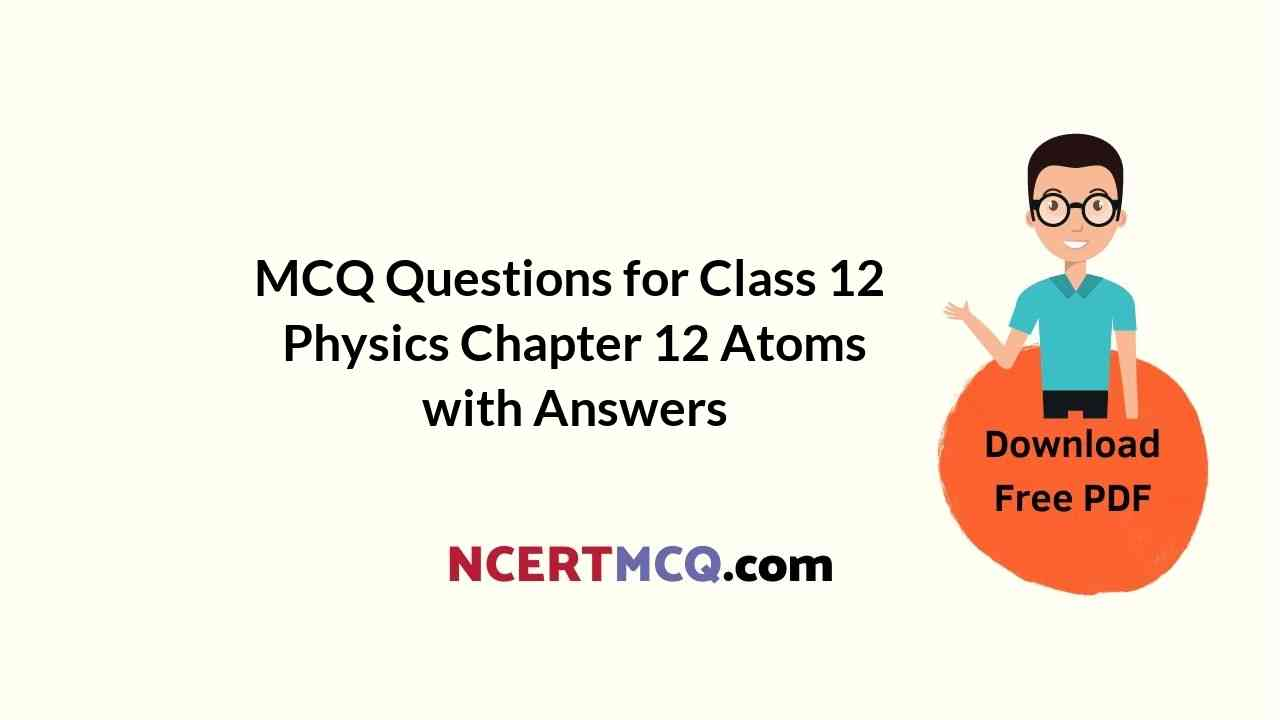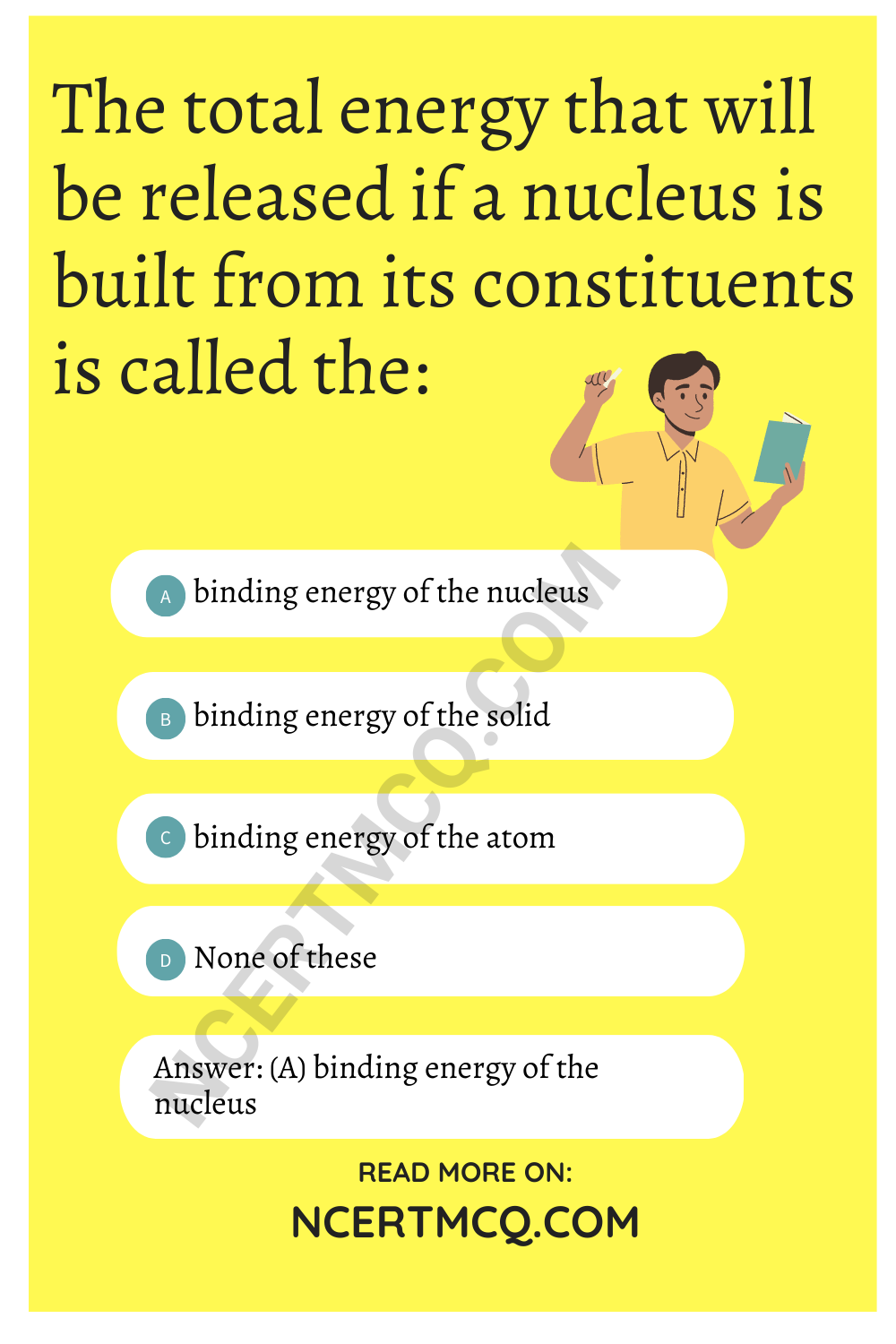Check the below NCERT MCQ Questions for Class 12 Physics Chapter 12 Atoms with Answers Pdf free download. MCQ Questions for Class 12 Physics with Answers were prepared based on the latest exam pattern. We have provided Atoms Class 12 Physics MCQs Questions with Answers to help students understand the concept very well.

## Atoms Class 12 MCQs Questions with Answers

Atoms Class 12 MCQ Question 1.
An atom stays in an excited state for about:
(a) 10 micro seconds
(b) 10 milli seconds
(c) 10 nano seconds
(d) 10 seconds

Atoms MCQ Questions Class 12 Question 2.
The energy equivalent to one atomic mass unit is :
(a) 1.6 × 10-19 J
(b) 6.02 × 1023 J
(c) 9.31 MeV
(d) 931 MeV

Chapter 12 Physics Class 12 MCQs Question 3.
Who explained the splitting of special lines in magnetic field?
(a) Zeeman
(b) Bohr
(c) Summerfield
(d) Einstein

Class 12 Physics MCQ Question 4.
The mass of a neutron is:
(a) 1.00866 u
(b) 1.0866 u
(c) 1.866 u
(d) 0.1866 u

Physics Class 12 MCQ Questions Question 5.
Isobars have the same:
(a) A
(b) Z
(c) N
(d) All the aboveClass 12 MCQ Physics Question 6.
Isotones have the same:
(a) A
(b) Z
(c) N
(d) All the above

Physics 12th MCQ Questions Question 7.
Half-life of a substance depends on:
(a) pressure
(b) temperature
(c) density
(d) None of these

Learninsta Class 12 Physics Question 8.
The concept of electron spin was introduced by:
(a) Becquerel
(b) Goudsmit
(c) Millikan
(d) Uhlenbeek and Goudsmit

Atomic Physics Questions And Answers Pdf Question 9.
The principle that a quantum orbital cannot be occupied by more than two electrons was given by:
(a) Pauli
(b) Millikan
(c) Hund
(d) None of these

Physics MCQ Class 12 Question 10.
For ionization of excited Hydrogen atom, the, required energy is:
(a) a little less than 13.6
(b) equal to 13.6
(c) more than 13.6
(d) 3.4 or less

Physics Class 12 MCQ Question 11.
According to Bohr’s theory of hydrogen atom, the radius r of stationary orbit are related to principal quantum number n as:
(a) rg ∝ $$\frac{1}{n^2}$$
(b) rg ∝ $$\frac{1}{n}$$
(c) rg ∝ n
(d) rg ∝ n²

MCQ Physics Class 12 Question 12.
Fg and Fe represents the gravitational and electrostatic force respectively between two electrons situated at some distance the ratio $$\frac{F_g}{F_e}$$ is if the order of:
(a) 9.8
(b) 109
(c) 1042
(d) 10-42

Question 13.
(a) Joliot
(b) Becquerel
(c) Pauli
(d) None of these

Question 14.
P-decay produces:
(a) isobars
(b) isotopes
(c) isotones
(d) All the above

Question 15.
(a) Joliot
(b) Becquerel
(c) Pauli
(d) None of these

Question 16.
Transmutation of nuclei was discovered by:
(a) Rutherford
(b) Becquerel
(c) Pauli
(d) None of these

Question 17.
The energy equivalent to mass defect is called :
(a) binding energy
(b) internal energy
(c) external energy
(d) enthalpy

Question 18.
The total energy that will be released if a nucleus is built from its constituents is called the:
(a) binding energy of the nucleus
(b) binding energy of the solid
(c) binding energy of the atom
(d) None of these

Answer: (a) binding energy of the nucleusQuestion 19.
The binding energy of $$_{2}^{4}$$He is about:
(a) 28.3 eV
(b) 28.3 MeV
(c) 28.3 J
(d) 2.83 MeV

Question 20.
The binding energy of a deuteron is about:
(a) 2.22 MeV
(b) 2.22 J
(c) 2.22 eV
(d) None of these